# IR measurement/compensation

Smart Manager uses 2 methods to measure IR value. Measured IR value is recorded on AuxV3|IR data.

Note: If you set parameters for IR measurement, Smart manager software can NOT read AuxV3 value.

## 1.       Current Interrupt method

You can measure IR value using Potentiostatic IR measurement technique menu on basic techniques.

This will apply potential shortly <3msec and stop current flowing using FET switch. Smart software measure voltage and current before and after FET switch on.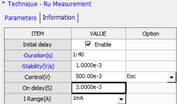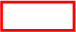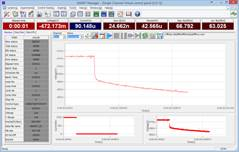Measured data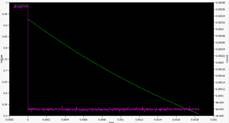Ø  Linear fitting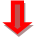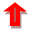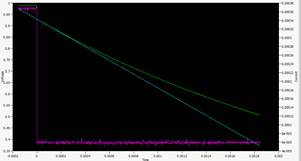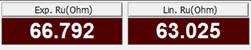Ø  Exponential fitting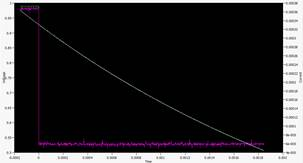Software read raw data using 2usec sampling rate and calculate Ru using linear fitting and Exponential fitting automatically and shows these value at ROI value as the above.

You can measure IR value using Galvanostatic IR measurement technique menu on basic techniques.

This will apply current shortly <3msec and stop current flowing using FET switch. Smart software measure voltage and current before and after FET switch on.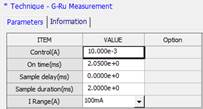## 2.       Positive feedback IR compensation

You can use IR value, which you got current interrupt IR measurement technique, for positive feedback IR compensation.

You can select “P-feedback” for “IR Comp” and input IR value (ohm) on “PF IR (ohm)”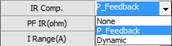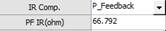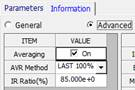If you input 80% compensation and 100Ohm for PF IR value, SmartManager will compensate 80ohm (100Ohm x80%).  SM software read current value and calculate IR drop using 80ohm (If measured current is 1mA then 80Ohm x 1mA= 80mV will be added to control voltage to compensate IR drop.

## 3.       Dynamic Method

You can measure IR value during experiment. Smart Manager Software can support this function using fixed frequency impedance measurement.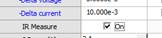If you do not know frequency value for IR, you need to run scanning frequency EIS measurement in PEIS or GEIS at high frequency range and determine frequency value at Zimg is zero value.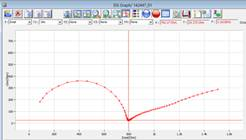.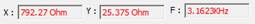From the above eis spectra, IR value is 792ohm and frequency for IR is 3.16 kHz

Dynamic IR measurement will work per each control step. If control step is constant current, dynamic IR measurement will do fixed frequency Galvanostatic EIS and if control step is constant potential, dynamic IR measurement will do fixed frequency Potentiostatic EIS at starting point.

Parameter setup for dynamic IR measurement is on “advanced tab” in device utility menu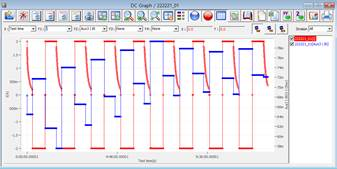I, IR vs. time

CC/CV charging CC discharging with dynamic IR measurement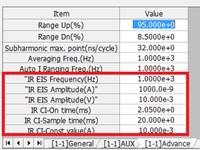l  IR EIS frequency (Hz): frequency value for dynamic IR measurement. Unit is Hz.

l  IR EIS amplitude (A): amplitude for constant current control. Unit is A (e.g. for 10mA, input 10m). Bias current is 0A.

l  IR EIS amplitude (V): Amplitude for constant potential control. Unit is V (e.g. for 5mV, input 5m). Bias voltage is Eoc.

l  IR CI On time (ms): On rest duration, Current interrupt On time: Unit is msec *1

l  IR CI Sample time(ms): Current interrupt sample delay time: Unit is msec *1

l  IR CI const value(A): On rest duration, Galvanostatic On current *1

*1: For future function.

Using this function, you do not need to input PF IR value manually. SmartManager will measure IR value using dynamic IR measurement and compensate measured IR value.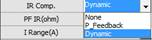You can set IR compensation rate on “information tab”-“Advanced”. Default value is 85%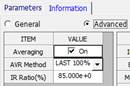If smart manager get IR vale as 100 ohm with dynamic IR measurement and if you input 80% compensation then SmartManager will compensate 80ohm (100Ohm x80%).  SM software read current value and calculate IR drop using 80ohm (If measured current is 1mA then 80Ohm x 1mA= 80mV will be added to control voltage to compensate IR drop)

## 4.       Compensable Max IR value.

Compensable maximum IR value for IR compensation is depending on sensing resistor for each current range.

It cannot exceed 1000% of Rs (Sensing resistor)

If input Ru value for positive feedback or measured IR value by dynamic IR measurement is larger than 10xRs value, Potentiostat will oscillate.

 Model SP5/MP5 SP2/MP2/BP2 SP1/MP1/PP1e Rs(Sensing resistor value) Max Ru value I range 5A 2A 1A 100mOhm 1Ohm 500mA 200mA 100mA 1Ohm 10Ohm 50mA 20mA 10mA 10Ohm 100Ohm 5mA 2mA 1mA 100Ohm 1kOhm 500uA 200uA 100uA 1kOhm 10kOhm 50uA 20uA 10uA 10kOhm 100kOhm 5uA 2uA 1uA 100kOhm 1MOhm 500nA 200nA 100nA 1MOhm 10Mohm 50nA 20nA 10Mohm 100MOhm 5nA 2nA 100MOhm 1GOhm

## 5.       IR measurement only in techniques

You can enable/disable IR measurement for the following techniques

1.        Basic  package (Current Interrupt Method)

A.         Potentiostatic Ru measurement

B.         Galvanostatic Ru measurement

2.        Energy package (Dynamic Method)

A.         CC/CV test

B.         CC/CC test

C.         EVS test

D.         Variable scan rate CV

E.         Pstat IV curve

F.         Gstat IV curve

## 6.       IR measurement and compensation in techniques

You can enable/disable IR compensation only (positive feedback) or IR measurement/IR compensation (dynamic) for the following techniques

1.        Basic techniques (Positive feedback)

A.         Potentiostatic

B.         Potential sweep

C.         Cyclic voltammetry

2.        Corrosion techniques (positive feedback or dynamic)

A.         Tafel

B.         Potentiodynamic

C.         Cyclic polarization

D.         polarization resistance

E.         RpEc trend

F.         Reactivation potential

3.        Echem analysis package (positive feedback)

A.         Chronoamperometry

B.         Chronocoulometry

C.         Normal pulse voltammetry

D.         Differential pulse voltammetry

E.         Reverse normal pulse voltammetry

F.         Square wave voltammetry

G.        Linear sweep voltammetry

H.        Sampled DC voltammetry